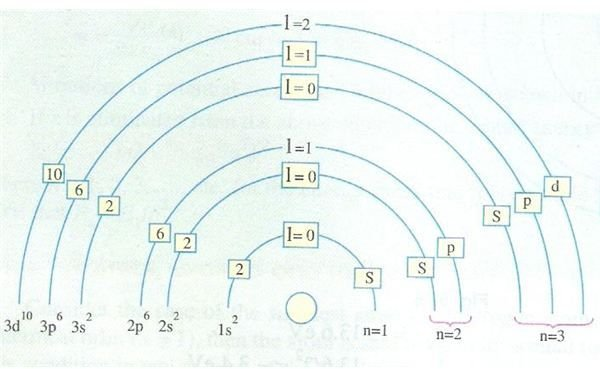# Four quantum numbers explained: Learn about the atomic structure of matter

## Introduction

We have been studying about the Bohr’s atomic model and the energy bands associated with it. In this article I would like to discuss a few theoretical aspects related to the model of the atom which would be very useful for anyone interested in knowing further about digital electronics. We will study the main four quantum numbers as described in electrical theory and their significance.

## Principal Quantum Number

We know from our study of the Bohr’s model that the electrons can occupy only discrete energy levels which are given by the equation mvr = nh/2π where n >= 1. This is also known as the principal quantum number and it gives the values that the various orbital radii can take.

I would also like to mention about the three states of an electron at this stage. These stages are

• Normal
• Excited
• Ionized

A normal electron is obviously in the normal state, while it gets excited and goes in higher orbits upon receiving energy. If it gains too much and gets dissociated with the atom, then the atom is in an ionized state.

## Azimuthal Quantum Number

It was observed after initial Bohr’s hypothesis that in actual practice, the orbits defined by the principal quantum number are not single orbits but each orbit contains one or more sub-orbits. Hence a new term came into being and these group of sub-orbits related to a particular orbit are collectively called a shell and there are sub-shells within the shell.

If this seems bit confusing just take a look at the adjoining diagram and you will see what I exactly mean. The picture beautifully depicts the various shells, sub-shells and their nomenclature. The shells are known as K, L, M and so on.K stands for shell given by n = 1 and has not sub-shell (or you can say it is a sub-shell of itself)

L stands for shell given by n = 2 and has two sub-shells

……………….

The symbol for azimuthal quantum number is the letter “l (small L)”

## Magnetic Quantum Number

This is the third quantum number and denoted by the symbol ml and it gives the spatial orientation of the elliptical orbits. For a given azimuthal quantum number “l”, the corresponding value of ml can be from –l to +l. Hence in another words it can be said to describe the number of sub-sub-shells of electron orbits.

## Spin Quantum Number

Last but not least, the ms or magnetic spin quantum number gives the orientation of the electron spin axis and can have only two values of +1/2 and -1/2.

In our next article we will study a very interesting phenomenon namely Pauli’s exclusion principle and well as the distribution of electrons within the atom.

## References

Digital Systems: Principles & Applications by Ronald. J. Tocci